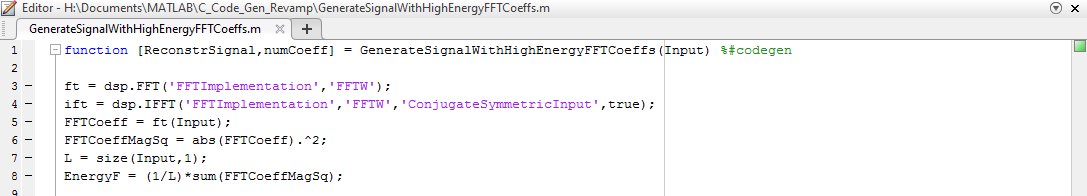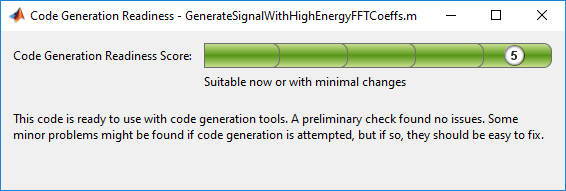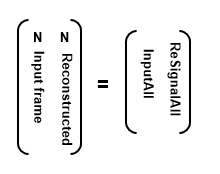## Generate C Code from MATLAB Code

MATLAB® Coder™ generates highly optimized ANSI® C and C++ code from functions and System objects in Communications Toolbox™ . You can deploy this code in a wide variety of applications. The workflow described in this topic uses DSP System Toolbox™ features but the same workflow applies for Communications Toolbox.

This example generates C code from the Construct a Sinusoidal Signal Using High Energy FFT Coefficients example and builds an executable from the generated code.

Here is the MATLAB code for this example:

```L = 1020; Sineobject = dsp.SineWave('SamplesPerFrame',L,... 'PhaseOffset',10,'SampleRate',44100,'Frequency',1000); ft = dsp.FFT('FFTImplementation','FFTW'); ift = dsp.IFFT('FFTImplementation','FFTW','ConjugateSymmetricInput',true); rng(1); numIter = 1000; for Iter = 1:numIter Sinewave1 = Sineobject(); Input = Sinewave1 + 0.01*randn(size(Sinewave1)); FFTCoeff = ft(Input); FFTCoeffMagSq = abs(FFTCoeff).^2; EnergyFreqDomain = (1/L)*sum(FFTCoeffMagSq); [FFTCoeffSorted, ind] = sort(((1/L)*FFTCoeffMagSq),1,'descend'); CumFFTCoeffs = cumsum(FFTCoeffSorted); EnergyPercent = (CumFFTCoeffs/EnergyFreqDomain)*100; Vec = find(EnergyPercent > 99.99); FFTCoeffsModified = zeros(L,1); FFTCoeffsModified(ind(1:Vec(1))) = FFTCoeff(ind(1:Vec(1))); ReconstrSignal = ift(FFTCoeffsModified); end max(abs(Input-ReconstrSignal)) plot(Input,'*'); hold on; plot(ReconstrSignal,'o'); hold off;```

You can run the generated executable inside the MATLAB environment. In addition, you can package and relocate the code to another development environment that does not have MATLAB installed. You can generate code using the MATLAB Coder app or the `codegen` (MATLAB Coder) function. This example shows you the workflow using the `codegen` function. For more information on the app workflow, see Generate C Code by Using the MATLAB Coder App (MATLAB Coder).

### Set Up the Compiler

The first step is to set up a supported C compiler. MATLAB Coder automatically locates and uses a supported installed compiler. You can change the default compiler using `mex -setup`. For more details, see Change Default Compiler. For a current list of supported compilers, see Supported and Compatible Compilers.

### Break Out the Computational Part of the Algorithm into a MATLAB Function

To generate C code, the entry point must be a function. You do not have to generate code for the entire MATLAB application. If you have specific portions that are computationally intensive, generate code from these portions in order to speed up your algorithm. The harness or the driver that calls this MATLAB function does not need to generate code. The harness runs in MATLAB and can contain visualization and other verification tools that are not actually part of the system under test. For example, in the Construct a Sinusoidal Signal Using High Energy FFT Coefficients example, the `plot` functions plot the input signal and the reconstructed signal. `plot` is not supported for code generation and must stay in the harness. To generate code from the harness that contains the visualization tools, rewrite the harness as a function and declare the visualization functions as extrinsic functions using `coder.extrinsic` (MATLAB Coder). To run the generated code that contains the extrinsic functions, you must have MATLAB installed on your machine.

The MATLAB code in the `for` loop that reconstructs the original signal using high-energy FFT coefficients is the computationally intensive portion of this algorithm. Speed up the `for` loop by moving this computational part into a function of its own, `GenerateSignalWithHighEnergyFFTCoeffs.m`.

```L = 1020; Sineobject = dsp.SineWave('SamplesPerFrame',L,... 'SampleRate',44100,'Frequency',1000); rng(1); numIter = 1000; for Iter = 1:numIter Sinewave1 = Sineobject(); Input = Sinewave1 + 0.01*randn(size(Sinewave1)); [ReconstrSignal,numCoeff] = GenerateSignalWithHighEnergyFFTCoeffs(Input); end max(abs(Input-ReconstrSignal)) figure(1); plot(Input) hold on; plot(ReconstrSignal,'*') hold off ```
```function [ReconstrSignal,numCoeff] = GenerateSignalWithHighEnergyFFTCoeffs(Input) ft = dsp.FFT('FFTImplementation','FFTW'); ift = dsp.IFFT('FFTImplementation','FFTW','ConjugateSymmetricInput',true); FFTCoeff = ft(Input); FFTCoeffMagSq = abs(FFTCoeff).^2; L = size(Input,1); EnergyF = (1/L)*sum(FFTCoeffMagSq); [FFTCoeffSorted, ind] = sort(((1/L)*FFTCoeffMagSq),1,'descend'); CumFFTCoeffs = cumsum(FFTCoeffSorted); EnergyPercent = (CumFFTCoeffs/EnergyF)*100; Vec = find(EnergyPercent > 99.99); FFTCoeffsModified = zeros(L,1); FFTCoeffsModified(ind(1:Vec(1))) = FFTCoeff(ind(1:Vec(1))); numCoeff = Vec(1); ReconstrSignal = ift(FFTCoeffsModified); end ```

### Make Code Suitable for Code Generation

Before you generate code, you must prepare your MATLAB code for code generation.

#### Check Issues at Design Time

The first step is to eliminate unsupported constructs and check for any code generation issues. For a list of Communications Toolbox features supported by MATLAB Coder, see Functions and System Objects Supported for C Code Generation. For a list of supported language constructs, see MATLAB Language Features Supported for C/C++ Code Generation (MATLAB Coder).

The code analyzer detects coding issues at design time as you enter the code. To enable the code analyzer, you must add the `%#codegen` pragma to your MATLAB file.The code generation readiness tool screens MATLAB code for features that are not supported for code generation. One of the ways to access this tool is by right-clicking on the MATLAB file in its current folder. Running the code generation tool on `GenerateSignalWithHighEnergyFFTCoeffs.m` finds no issues.#### Check Issues at Code Generation Time

Before you generate C code, ensure that the MATLAB code successfully generates a MEX function. The `codegen` (MATLAB Coder) command used to generate the MEX function detects any errors that prevent the code for being suitable for code generation.

Run `codegen` on `GenerateSignalWithHighEnergyFFTCoeffs.m` function.

`codegen -args {Input} GenerateSignalWithHighEnergyFFTCoeffs `

The following message appears in the MATLAB command prompt:

```??? The left-hand side has been constrained to be non-complex, but the right-hand side is complex. To correct this problem, make the right-hand side real using the function REAL, or change the initial assignment to the left-hand side variable to be a complex value using the COMPLEX function. Error in ==> GenerateSignalWithHighEnergy Line: 24 Column: 1 Code generation failed: View Error Report Error using codegen ```

This message is referring to the variable `FFTCoeffsModified`. The coder is expecting this variable to be initialized as a complex variable. To resolve this issue, initialize the `FFTCoeffsModified` variable as complex.

`FFTCoeffsModified = zeros(L,1)+0i;`

Rerun the `codegen` function and you can see that a MEX file is generated successfully in the current folder with a `.mex` extension.

`codegen -args {Input} GenerateSignalWithHighEnergyFFTCoeffs `

#### Check Issues at Run Time

Run the generated MEX function to see if there are any run-time issues reported. To do so, replace

`[ReconstrSignal,numCoeff] = GenerateSignalWithHighEnergyFFTCoeffs(Input);`
with
`[ReconstrSignalMex,numCoeffMex] = GenerateSignalWithHighEnergyFFTCoeffs_mex(Input);`
inside the harness.

The harness now looks like:

```L = 1020; Sineobject = dsp.SineWave('SamplesPerFrame',L,... 'SampleRate',44100,'Frequency',1000); rng(1); numIter = 1000; for Iter = 1:numIter Sinewave1 = Sineobject(); Input = Sinewave1 + 0.01*randn(size(Sinewave1)); [ReconstrSignalMex,numCoeffMex] = GenerateSignalWithHighEnergyFFTCoeffs_mex(Input,L); end max(abs(Input-ReconstrSignalMex)) figure(1); plot(Input) hold on; plot(ReconstrSignalMex,'*') hold off ```

The code runs successfully, indicating that there are no run-time errors.

### Compare the MEX Function with the Simulation

Notice that the harness runs much faster with the MEX function compared to the regular function. The reason for generating the MEX function is not only to detect code generation and run-time issues, but also to speed up specific parts of your algorithm. For an example, see Signal Processing Algorithm Acceleration in MATLAB.

You must also check that the numeric output results from the MEX and the regular function match. Compare the reconstructed signal generated by the `GenerateSignalWithHighEnergyFFTCoeffs.m` function and its MEX counterpart `GenerateSignalWithHighEnergyFFTCoeffs_mex`.

```max(abs(ReconstrSignal-ReconstrSignalMex)) ans = 2.2204e-16```

The results match very closely, confirming that the code generation is successful.

### Generate a Standalone Executable

If your goal is to run the generated code inside the MATLAB environment, your build target can just be a MEX function. If deployment of code to another application is the goal, then generate a standalone executable from the entire application. To do so, the harness must be a function that calls the subfunction `GenerateSignalWithHighEnergyFFTCoeffs`. Rewrite the harness as a function.

```function reconstructSignalTestbench() L = 1020; Sineobject = dsp.SineWave('SamplesPerFrame',L,... 'SampleRate',44100,'Frequency',1000); rng(1); numIter = 1000; for Iter = 1:numIter Sinewave1 = Sineobject(); Input = Sinewave1 + 0.01*randn(size(Sinewave1)); [ReconstrSignal,numCoeff] = GenerateSignalWithHighEnergyFFTCoeffs(Input,L); end```

Log all 1000 frames of the input and reconstructed signal and the number of FFT coefficients used to reconstruct each frame of the signal. Write all this data to a binary file named `data.bin` using the `dsp.BinaryFileWriter` System object™. This example logs the number of coefficients, which are scalar values, as the first element of each frame of the input signal and the reconstructed signal. The data to be written has a frame size of M = L + 1 and has a format that looks like this figure.N is the number of FFT coefficients that represent 99.99% of the signal energy of the current input frame. The meta data of the binary file specifies this information. Release the binary file writer and close the binary file at the end.

The updated harness function, `reconstructSignalTestbench`, is shown here:

```function reconstructSignalTestbench() L = 1020; Sineobject = dsp.SineWave('SamplesPerFrame',L,... 'SampleRate',44100,'Frequency',1000); header = struct('FirstElemInBothCols','Number of Coefficients',... 'FirstColumn','Input','SecondColumn','ReconstructedSignal'); bfw = dsp.BinaryFileWriter('data.bin','HeaderStructure',header); numIter = 1000; M = L+1; ReSignalAll = zeros(M*numIter,1); InputAll = zeros(M*numIter,1); rng(1); for Iter = 1 : numIter Sinewave1 = Sineobject(); Input = Sinewave1 + 0.01*randn(size(Sinewave1)); [ReconstrSignal,numCoeffs] = GenerateSignalWithHighEnergyFFTCoeffs(Input); InputAll(((Iter-1)*M)+1:Iter*M) = [numCoeffs;Input]; ReSignalAll(((Iter-1)*M)+1:Iter*M) = [numCoeffs;ReconstrSignal]; end bfw([InputAll ReSignalAll]); release(bfw); ```

The next step in generating a C executable is to create a `coder.config` object for an executable and provide a `main.c` function to this object.

```cfg = coder.config('exe'); cfg.CustomSource = 'reconstructSignalTestbench_Main.c';```

Here is how the `reconstructSignalTestbench_Main.c` function looks for this example.

```/* ** reconstructSignalTestbench_main.c * * Copyright 2017 The MathWorks, Inc. */ #include <stdio.h> #include <stdlib.h> #include "reconstructSignalTestbench_initialize.h" #include "reconstructSignalTestbench.h" #include "reconstructSignalTestbench_terminate.h" int main() { reconstructSignalTestbench_initialize(); reconstructSignalTestbench(); reconstructSignalTestbench_terminate(); return 0; } ```

For additional details on creating the main function, see Generating Standalone C/C++ Executables from MATLAB Code (MATLAB Coder).

Set the `CustomInclude` property of the configuration object to specify the location of the main file. In this example, the location is the current folder.

`cfg.CustomInclude = ['"',pwd,'"'];`

Generate the C executable by running the following command in the MATLAB command prompt:

`codegen -config cfg -report reconstructSignalTestbench`

MATLAB Coder compiles and links the main function with the C code that it generates from the `reconstructSignalTestbench.m`.

If you are using Windows, you can see that `reconstructSignalTestbench.exe` is generated in the current folder. If you are using Linux, the generated executable does not have the `.exe` extension.

### Read and Verify the Binary File Data

Running the executable creates a binary file, `data.bin`, in the current directory and writes the input, reconstructed signal, and the number of FFT coefficients used to reconstruct the signal.

`!reconstructSignalTestbench`

You can read this data from the binary file using the `dsp.BinaryFileReader` object. To verify that the data is written correctly, read data from the binary file in MATLAB and compare the output with variables `InputAll` and `ReSignalAll`.

The header prototype must have a structure similar to the header structure written to the file. Read the data as two channels.

```M = 1021; numIter = 1000; headerPro = struct('FirstElemInBothCols','Number of Coefficients',... 'FirstColumn','Input','SecondColumn','ReconstructedSignal'); bfr = dsp.BinaryFileReader('data.bin','HeaderStructure',... headerPro,'SamplesPerFrame',M*numIter,'NumChannels',2); Data = bfr(); ```

Compare the first channel with `InputAll` and the second channel with `ReSignalAll`.

`isequal(InputAll,Data(:,1))`
```ans = logical 1```
```isequal(ReSignalAll,Data(:,2)) ```
```ans = logical 1```

The results match exactly, indicating a successful write operation.

### Relocate Code to Another Development Environment

Once you generate code from your MATLAB algorithm, you can relocate the code to another development environment, such as a system or an integrated development environment (IDE) that does not include MATLAB. You can package the files into a compressed file using the `packNGo` function at the command line or the Package option in the MATLAB Coder app. For an example that illustrates both the workflows, see Package Code for Other Development Environments (MATLAB Coder). For more information on the `packNGo` option, see `packNGo` in RTW.BuildInfo Methods (MATLAB Coder). You can relocate and unpack the compressed zip file using a standard zip utility. For an example on how to package the executable generated in this example, see Relocate Code Generated from MATLAB Code to Another Development Environment.